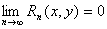3．函数的幂级数展开式

[幂级数的唯一性定理]  如果函数f(x)(f(x,y))x=0(x=0,y=0)可以展开成幂级数

f(x)=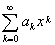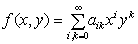[幂级数的存在性定理]

若函数f (x)x=0具有任意阶导数，且当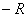xR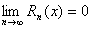若函数f (x,y)在点(0,0)具有任意阶偏导数，且当(x,y)xy平面上某一区域M上的点时